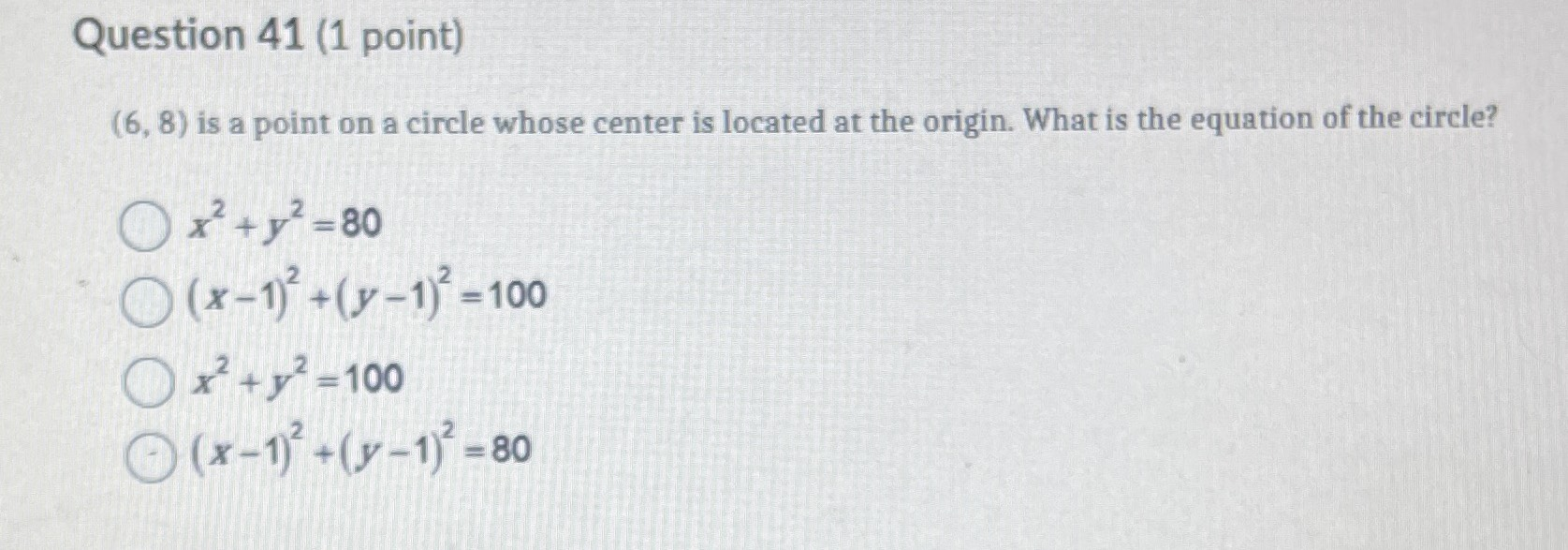### ¿Todavía tienes preguntas de matemáticas?

Pregunte a nuestros tutores expertos
Algebra
Pregunta$$( 6,8 )$$ is a point on a circle whose center is located at the origin. What is the equation of the circle?

$$x ^ { 2 } + y ^ { 2 } = 80$$

$$( x - 1 ) ^ { 2 } + ( y - 1 ) ^ { 2 } = 100$$

$$x ^ { 2 } + y ^ { 2 } = 100$$

$$( x - 1 ) ^ { 2 } + ( y - 1 ) ^ { 2 } = 80$$